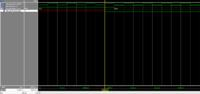# Describe in VHDL a generator parallel 4 bits to serial 1 bit

Status
Not open for further replies.

#### isamel85

##### Newbie level 6
Hello,
I want to describe in VHDL a generator parallel 4 bits to serial 1 bit.
Indeed, at each clock edge (250 kHz), we take only one bit starting with the least significant bit (LSB).
Example:
Input = "0101" (over 4 bits)
So at first clock edge, output = '1' (LSB)
Second clock edge, output = '0'
Third clock edge, output = '1'
Fourth clock edge, output = '0' (MSB)
There's someone there who can help me?
And thank you

Ignoring all the declarations and the other verbose stuff...

Code VHDL - [expand]1
2
3
4
5
6
7
8
9
10
11
process (clk)
begin
if rising_edge(clk) then
sr <= parallel_data;
else
sr <= '0' & sr(3 downto 1);
end
end if;
end process;
lsb_out <= sr(0);

I tried it but it still gives me a single output state (either 0 or 1) even if I have a new rising edge, the value remains the same

Code VHDL - [expand]1
2
3
4
5
6
7
8
9
10
11
12
13
14
15
16
17
18
19
20
21
22
23
24
25
26
27
28
29
30
31
library IEEE;
use ieee.std_logic_1164.all;
use ieee.numeric_std.all;

entity generator_bits is
port ( Output_data      : out  std_logic;
Clock_250kHz        : in std_logic;
Input_datas : in std_logic_vector(3 downto 0)
);

end generator_bits;

architecture Behavioral of generator_bits is
signal  register_temp : std_logic_vector (3 downto 0);
begin
process (Clock_250kHz)

begin
if rising_edge(Clock_250kHz) then
register_temp <= Input_datas;
else
register_temp <= '0' & register_temp(3 downto 1);
end if;

end if;
end process;
Output_data <= register_temp(0);

end Behavioral;

Last edited by a moderator:

Wrong testbench?

I tried it but it still gives me a single output state (either 0 or 1) even if I have a new rising edge, the value remains the same

This is my test bench:
Code:
LIBRARY ieee;
USE ieee.std_logic_1164.ALL;

ENTITY test_gen IS
END test_gen;

ARCHITECTURE behavior OF test_gen IS

-- Component Declaration for the Unit Under Test (UUT)

COMPONENT generateur_bits
PORT(
Output_data : OUT  std_logic;
Clock_250kHz : IN  std_logic;
Input_datas : IN  std_logic_vector(3 downto 0)
);
END COMPONENT;

--Inputs
signal Clock_250kHz : std_logic := '0';
signal Load_sr : std_logic := '0';
signal Input_datas : std_logic_vector(3 downto 0) := (others => '0');

--Outputs
signal Output_data : std_logic;

-- Clock period definitions
constant Clock_250kHz_period : time := 4 us;

BEGIN

-- Instantiate the Unit Under Test (UUT)
uut: generateur_bits PORT MAP (
Output_data => Output_data,
Clock_250kHz => Clock_250kHz,
Input_datas => Input_datas
);

-- Clock process definitions
Clock_250kHz_process :process
begin
Clock_250kHz <= '0';
wait for Clock_250kHz_period/2;
Clock_250kHz <= '1';
wait for Clock_250kHz_period/2;
end process;

-- Stimulus process
stim_proc: process
begin
-- hold reset state for 100 ns.
--wait for 16 us;

wait for Clock_250kHz_period*4;
Input_datas <= "1100";
-- insert stimulus here

wait;
end process;

END;
Show please the result of simulation#### Attachments

•Image3.png
81.4 KB · Views: 44

Data is shifted when "Load_sr" is '0' and loaded when it is '1'.
You must put it back to '0' after loading the parallel data.

Status
Not open for further replies.